# Guppies

Audrey has some guppies in a fish tank. The ration of the oranges guppies to silver guppies is 3:5. She has 12y oranges guppies. Write the number of silver guppies she has in terms of y

Result

s = (Correct answer is: 20y)### Step-by-step explanation:Did you find an error or inaccuracy? Feel free to write us. Thank you!Tips to related online calculators
Check out our ratio calculator.

## Related math problems and questions:

• Unknown number 5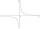Daniel thinks an integer. When he change this number at a ratio of 2:5, he got the number 2.8. Determine what number think Daniel.
• The perimeter 3The perimeter of a rectangle is 35 cm. The ratio of the length to its width is 3:2. Calculate the dimensions of the rectangle
• Babysitting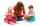The amount that Susan charges per hour for babysitting are directly proportional to the number of children she is watching. She charges \$4.50 for 3 kids. How much would she charge to babysit 5 kids?
• Ratio three numbers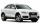Three numbers SUV are in the ratio 1:2:3. Their sum is 24. Find this numbers and write their add and sum.
• A cylindrical tankA cylindrical tank can hold 44 cubic meters of water. If the radius of the tank is 3.5 meters, how high is the tank?
• Natural gas in kWh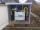Gas consumption for 2017 was 11,301 kWh I paid 532 € How much 1 m3?
• Ratio6 numbers are in the ratio 1:5:1:5:5:5. Their sum is 242. What are the numbers?
• Scrapbook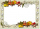Jo is making a scrapbook for her best friend’s 5th grade graduation. She used 72 photos with 6 photos on each page. Jo had to remove two of the photos on each page in order to make room for the writing she wanted to include. Write an expression represents
• Moneys in triple ratioMilan, John and Lili have a total 344 euros. Their amounts are in the ratio 1:2:5. Determine how much each of them has?
• Divide moneyDivide 1200 USD at a ratio of 1:2:3:4:5:6:9:10
• Oranges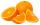Mother divided her three children's oranges in a ratio of 6:5:4. Two children gave 45 oranges. How many oranges were there?
• When 5When 5 is subtracted from both my numerator and denominator I become 3/4. What fraction i am?
• BuildingAt the building, we divided 240 boards into two piles in a 5: 3 ratio. How many were fewer boards in the lower pile?
• StudyFor 7 hours of study, she scored a 75, so if she made 93, how many hours would she need to study?
• Ratio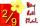Write the ratio with other numbers so that the value is the same: 2: 9
• Change the numbers in the ratio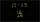Change the numbers 29, 38, and 43 in a 3: 4 ratio.
• Tank B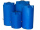Tank B has the same volume as Tank A. The volume of one section of Tank B is 4545 cubic feet. What is the volume, in cubic feet, of the other section of Tank B? Total volume of both tanks A+B is 15000 ft3. Enter your answer in the box.